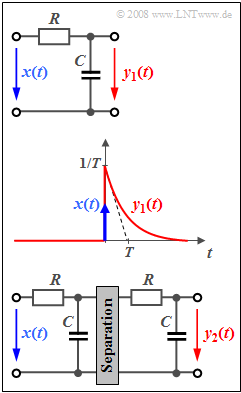# Exercise 1.4: Low-Pass Filter of 2nd OrderLow-pass filter of first order (top)
and of second order (bottom)

In  Exercise 1.1  and  Exercise 1.1Z  of the chapter  System Description in Frequency Domain  the so-called  "RC low-pass filters"  were described in the frequency domain.  Now, the time domain representation is elaborated on.

The circuit with the input signal  $x(t)$  and the output signal  $y_1(t)$  is a low-pass filter of first order with frequency response

$$H_{\rm 1}(f) = \frac{1}{1+{\rm j}\cdot f/f_{\rm 0}}.$$

Here,  $f_0 = 1/(2πRC)$  indicates the 3dB cut-off frequency.  If a Dirac-shaped signal  $x(t) = δ(t)$  is applied to the input, the signal  $y_1(t)$  is given by the system output according to the sketch in the middle.

The relationship between the system parameters  $R$  (resistance),  $C$  (capacitance)   and  $T$  is (see  Exercise 1.3Z):

$$T = \frac{1}{\omega_{\rm 0}}= \frac{1}{2\pi f_{\rm 0}} = R \cdot C.$$

For numerical calculations   $T = 1 \ \rm ms$  shall be used in the following.

The circuit below with input  $x(t)$  and output  $y_2(t)$  represents a low-pass filter of second order:

$$H_{\rm 2}(f) = \big[H_{\rm 1}(f)\big]^2 =\frac{1}{(1+{\rm j}\cdot f/f_{\rm 0})^2}.$$
• The impulse response associated with  $H_2(f)$  is  $h_2(t)$.
• The system parameter  $f_0$  no longer indicates the 3dB cut-off frequency for a low-pass filter of second or higher order.
• Furthermore, it should be noted that the two RC elements must be decoupled in order to achieve impedance matching.
• An operational amplifier, for example, is suitable for this.  However, this hint is not relevant for the solution of this task.

$$\int u \cdot {\rm e}^{a \cdot \hspace{0.03cm} u} \hspace{0.1cm}{\rm d}u = \frac{{\rm e}^{\hspace{0.03cm}a \cdot \hspace{0.03cm} u}}{a^2} \cdot (a \cdot u -1).$$

### Questions

1

State the impulse response  $h_1(t)$ .   At what time  $t_1$  has  $h_1(t)$  decreased to half of its maximum value?

 $t_1\ = \$ $\ \rm ms$

2

What is the output signal  $y_1(t)$  for  $x(t) = T · h_1(t)$?   What are the signal values at times  $t = 0$  and  $t = T$ ?

 $y_1(t = 0) \ = \$ $y_1(t = T) \ = \$

3

Compute the impulse response  $h_2(t)$  considering the result of  (2).   At what time  $t_2$  is  $h_2(t=t_2)$  maximum?

 $t_2 \ = \$  $\rm ms$ $h_2(t = t_2) \ = \$  $\rm 1/s$

4

What is the output signal  $y_2(t)$  if a step function  $x(t) = {\rm 2 \hspace{0.05cm}V} · γ(t)$  is applied to the input?   What are the signal values at  $t = T$  and  $t = 5T$ ?

 $y_2(t = T) \ = \$  $\rm V$ $y_2(t = 5T) \ = \$  $\rm V$

### Solution

#### Solution

(1)  By definition the impulse response is equal to the output signal if a Dirac delta function of weight  $1$  is applied to the input.

$$h_1(t) = y_1(t) ={1}/{T} \cdot {\rm e}^{-t/T}.$$
• For the time  $t_1$  the following shall hold:
$$h_1(t_{\rm 1}) ={1}/{T} \cdot {\rm e}^{-t_{\rm 1}/T} = \frac{1}{2T} \hspace{0.5cm}\Rightarrow\hspace{0.5cm}{t_{\rm 1}}/{T} = {\rm ln}(2)\hspace{0.1cm} \Rightarrow \hspace{0.1cm}t_{\rm 1} = 0.693 \cdot T \hspace{0.15cm}\underline{= {\rm 0.693\,\,ms}}.$$

(2)  The input signal  $x(t)$  is an exponentially decreasing impulse like the impulse response  $h_1(t)$  but dimensionless.

• Thus, according to the convolution theorem:
$$y_1(t) = x (t) * h_1 (t) = T \cdot \big[ h_1 (t) * h_1 (t) \big].$$
• The convolution is illustrated here for a specific time  $t$  by a sketch.
• The following is obtained after renaming the variables:
\begin{align*}h_1(\tau) & = \frac{1}{T} \cdot {\rm e}^{-\tau/T}\hspace{0.1cm} \Rightarrow \hspace{0.1cm}h_1(-\tau) = \frac{1}{T} \cdot {\rm e}^{\tau/T}\hspace{0.1cm} \Rightarrow \hspace{0.1cm}h_1(t-\tau) = \frac{1}{T} \cdot {\rm e}^{(-t+\tau)/T} \\ & \Rightarrow\hspace{0.5cm} y_1(t) = T \cdot \int\limits_{ - \infty }^{ + \infty } h_1 ( {t - \tau } )\cdot {h_1 ( \tau )} \hspace{0.1cm}{\rm d}\tau.\end{align*}
• For  $τ < 0$  the impulse response is  $h_1(τ) = 0$.   For  $τ > t$  the first convolution operand vanishes (see sketch). From this it follows that:
$$y_1(t) = T \cdot \frac{1}{T^2}\cdot \int_{ 0 }^{ t } {\rm e}^{(-t+\tau)/T} \cdot {\rm e}^{-\tau/T} \hspace{0.1cm}{\rm d}\tau = \frac{1}{T}\cdot \int_{ 0 }^{ t } {\rm e}^{-t/T} \hspace{0.1cm}{\rm d}\tau .$$
• Now,  it can be observed that the integrand is independent of the integration variable  $τ$.  Consequently, the following holds:
$$y_1(t) = {t}/{T} \cdot {\rm e}^{-t/T}\hspace{0.1cm} \Rightarrow \hspace{0.1cm} y_1(t =0) \hspace{0.15cm}\underline{=0}; \hspace{0.5cm}y_1(t =T)={\rm e}^{-1} \hspace{0.15cm}\underline{=0.368}.$$

(3)  Due to the interrelationship  $H_2(f) = H_1(f) · H_1(f)$  the following holds for the impulse response:   $h_2(t) = h_1 (t) * h_1 (t).$

• Except for the additional constant factor  $1/T$  the same result as in subtask  (2) is obtained:
$$h_2(t) ={t}/{T^2} \cdot {\rm e}^{-t/T}.$$
• The maximum value is obtained by setting the derivative to zero:
$$\frac{{\rm d} h_2(t)}{{\rm d}t} = \frac{1}{T^2} \cdot {\rm e}^{-t/T} \cdot \left( 1 - {t}/{T}\right) = 0 \hspace{0.5cm} \Rightarrow \hspace{0.5cm} t_{\rm 2} \hspace{0.15cm}\underline{= T = 1\,\,{\rm ms}} \hspace{0.5cm}\Rightarrow \hspace{0.5cm} h_2(t_{\rm 2}) =\frac{{\rm e}^{-1}}{T} =\frac{0.368}{1\,\,{\rm ms}} \hspace{0.15cm}\underline{ = {\rm 368 \hspace{0.1cm} 1/s}}.$$

(4)  In general or with the result from  (3)  the following applies to the step response:

$${\rm \sigma_2}(t) = \int_{ 0 }^{ t } {h_2 ( \tau )} \hspace{0.1cm}{\rm d}\tau = \frac{1}{T^2} \cdot \int_{ 0 }^{ t } \tau \cdot {\rm e}^{-\tau/T} \hspace{0.1cm}{\rm d}\tau .$$
• With the substitution  $u = τ/T$  and using the given integral it follows that:
$${\rm \sigma_2}(t) = \int_{ 0 }^{ t /T} u \cdot {\rm e}^{-u} \hspace{0.1cm}{\rm d}u ={\rm e}^{-u} \cdot (-u-1) |_{ 0 }^{ t /T} \hspace{0.5cm} \Rightarrow \hspace{0.5cm}{\rm \sigma_2}(t) = 1- \left( 1 + {t}/{T} \right) \cdot {\rm e}^{-t/T}.$$
• The following is obtained at the given times taking the factor  $2\ \rm V$ further into account:
$$y_2(t = T) = {\rm 2 \,V} \cdot \left( 1- 2 \cdot {\rm e}^{-1} \right) \hspace{0.15cm}\underline{= {\rm 0.528 \,V}}, \hspace{0.9cm}y_2(t = 5T) = {\rm 2 \,V} \cdot \left( 1- 6 \cdot {\rm e}^{-5} \right) \hspace{0.15cm}\underline{= {\rm 1.919 \,V}}.$$
• For even larger times  $y_2(t)$  approaches more and more the final value  $2\hspace{0.05cm} \rm V$ .